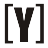# Kilowatts (kW) to Amps (A) Calculator

This Kilowatts to Amps calculator to convert power in Kilowatts into current result in Amps, select your current type (DC/AC), insert power in kW and voltage, then press Calculate to get the power result in Amps. Swap Amps to kW calculator.

 Select current type: DC (Direct Current) AC (Alternating Current) - Single phase AC (Alternating Current) - Three phase Enter power in kilowatts: kW Enter voltage in volts: V Current result in amps: A

### How to Calculate Kilowatts to Amps

AC, DC Kilowatts to Amps manual calculation

kW (DC) to Amps formula:

`I(A) = 1000 × P(kW) / V(V)`

current I in amps (A) is equal to 1000 multiplied by (P) power in kW, divided by the voltage V in volts.

kW (AC single phase) to Amps formula:

`I(A) = 1000 × P(kW) / (PF × V(V) )`

kW (AC three phase) to Amps formula:
Line to line voltage

`I(A) = 1000 × P(kW) / (√3 × PF × VL-L(V) )`

Line to neutral voltage

`I(A) = 1000 × P(kW) / (3 × PF × VL-N(V) )`

(PF) Power Factor values:

Device Typical power factorYoosFuhl.com Would you like to receive notifications on latest updates? No Yes